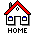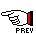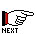# Introduction

The Unit Conversion for SansGUI utility program is a very small "simulator" program that is installed and run within the SansGUI Modeling and Simulation Environment. Although very small in its code size, this utility program fully exploits the unit conversion facility and features built into the SansGUI Run-Time Environment. Before we get started, let's introduce a couple of terms used in this program.

## Unit Dimension and Unit Category

A unit dimension describes the measurement of a dimension of quantity, such as length, mass, temperature, time, etc. It can also represent a compound dimension of quantity such as flow rate (mass per time or volume per time) and electric flux density (charge per area). Each unit dimension contains both SI and non-SI units in a table with their conversion factors. A unit category is a collection of unit dimensions used commonly in a particular field. Unit Conversion for SansGUI implements the following nine categories: 1) Space-Time, 2) Mechanics, 3) Heat, 4)Electricity, 5) Magnetism, 6) Radiology, 7) Light, 8) Substance, and 9) User.

Please consult the Unit Categories section for lists of unit dimensions they cover.

## Using Unit Conversion for SansGUI

The following sections cover the procedures of using Unit Conversion for SansGUI. It is recommended that you go through these sections sequentially to become familiar with this powerful unit conversion tool and take advantage of its capabilities.Unit Conversion for SansGUI Version 1.1